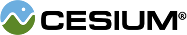#InterpolationAlgorithm

#### InterpolationAlgorithm() Core/InterpolationAlgorithm.js 17

The interface for interpolation algorithms.

### Members

#### staticCesium.InterpolationAlgorithm.type : String Core/InterpolationAlgorithm.js 23

Gets the name of this interpolation algorithm.

### Methods

#### staticCesium.InterpolationAlgorithm.getRequiredDataPoints(degree) → Number Core/InterpolationAlgorithm.js 32

Given the desired degree, returns the number of data points required for interpolation.
Name Type Description
`degree` Number The desired degree of interpolation.
##### Returns:
The number of required data points needed for the desired degree of interpolation.

#### staticCesium.InterpolationAlgorithm.interpolate(x, xTable, yTable, yStride, inputOrder, outputOrder, result) → Array.<Number> Core/InterpolationAlgorithm.js 67

Performs higher order interpolation. Not all interpolators need to support high-order interpolation, if this function remains undefined on implementing objects, interpolateOrderZero will be used instead.
Name Type Description
`x` Number The independent variable for which the dependent variables will be interpolated.
`xTable` Array.<Number> The array of independent variables to use to interpolate. The values in this array must be in increasing order and the same value must not occur twice in the array.
`yTable` Array.<Number> The array of dependent variables to use to interpolate. For a set of three dependent values (p,q,w) at time 1 and time 2 this should be as follows: {p1, q1, w1, p2, q2, w2}.
`yStride` Number The number of dependent variable values in yTable corresponding to each independent variable value in xTable.
`inputOrder` Number The number of derivatives supplied for input.
`outputOrder` Number The number of derivatives desired for output.
`result` Array.<Number> optional An existing array into which to store the result.
##### Returns:
The array of interpolated values, or the result parameter if one was provided.

#### staticCesium.InterpolationAlgorithm.interpolateOrderZero(x, xTable, yTable, yStride, result) → Array.<Number> Core/InterpolationAlgorithm.js 49

Performs zero order interpolation.
Name Type Description
`x` Number The independent variable for which the dependent variables will be interpolated.
`xTable` Array.<Number> The array of independent variables to use to interpolate. The values in this array must be in increasing order and the same value must not occur twice in the array.
`yTable` Array.<Number> The array of dependent variables to use to interpolate. For a set of three dependent values (p,q,w) at time 1 and time 2 this should be as follows: {p1, q1, w1, p2, q2, w2}.
`yStride` Number The number of dependent variable values in yTable corresponding to each independent variable value in xTable.
`result` Array.<Number> optional An existing array into which to store the result.
##### Returns:
The array of interpolated values, or the result parameter if one was provided.